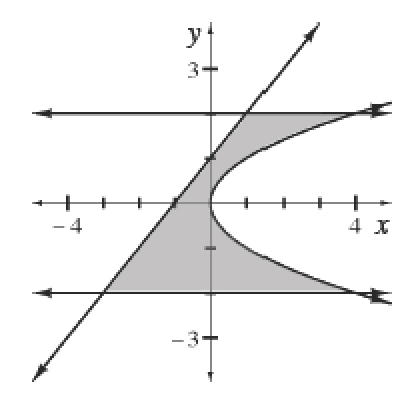### Home > APCALC > Chapter 6 > Lesson 6.4.1 > Problem6-119

6-119.

The graphs of the equations $x = y^2$, $x = y - 1$, $y = 2$, and $y = -2$ are shown at right. These four curves form the boundary of a region.

Show that the area of this region is $\frac { 28 } { 3 }$ $\text{un}^2$.
Hint: Use horizontal rectangles.$\text{Area between curves}=\int_{A}^{B}((\text{top function})-(\text{bottom function}))dy$

Since we are using horizontal rectangles, the infinitely small widths of each rectangle are measured on the $y$-axis, use $dy$ instead of $dx$.

The bounds:
Notice that BOTH equations are written with $y$ as the input and $x$ as the output.
Therefore, find the bounds of integration will be on the $y$-axis.
$A =$ lower $y$-value
$B =$ upper $y$-value

The integrand:
The 'top function' and 'bottom function' will also be determined with respect to the $y$-axis.
Let the 'top function' be the one with the right-most position, while the 'bottom function' will be the one with the 'left-most position.'
Another way to look at this is the 'top function' has the highest $x$-values, while the 'bottom function' has the lower $x$-values.